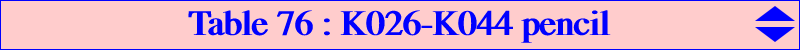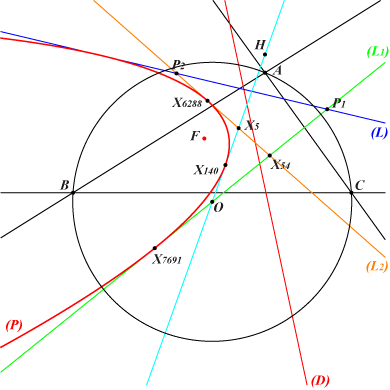K026 and K044 are two central circum-cubics with center X(5) passing through X(3), X(4) and the centers of the Johnson circles i.e. the reflections of A, B, C in X(5). They generate a pencil of central cubics passing through these same 9 points. One cubic decomposes into the Euler line and the circum-conic with center X(5). This is one of the three nKs of the pencil which also contains three psKs. One of them is in fact a pK namely K044. Every cubic (K) of the pencil meets the line at infinity and the circumcircle again at the same points as two isogonal pKs with pivots P1, P2 respectively. P1 lies on the line (L1) passing through X(i) for i = 3, 54, 97, 195, 1154, 1157, 1493, 1993, 2979, 5012, 5889, 5890, 6101, 6102, 6150, 7592, 7691, 8883, 10203, 10574, 10610, 10627, 11126, 11127, 11402, 11412, 11422, 11423, 12060, 12160, 12161, 12307, 12316, 12363, 12606, 13409, 13630, 15087, 15135, 15137, 15345, 15801, 16030, 16035, 16266, 16762, 18016, 19167, 19168, 19170, 19194, 19209, 19210, 19211, 20791, 22815, 23061, 23606, 25042, 25044, 27246, 31388, 31807, 31810, 32046, 32078, 32136, 32333, 32338, 32339, 32341, 32608, 34148, 34292, 34396, 34424, 34425, 34553, 34555, 34833, 35195, 35196, 35449. P2 lies on the line (L2) passing through X(i) for i = 5, 49, 54, 110, 265, 567, 1141, 3615, 4993, 6288, 7604, 8254, 8836, 8838, 8901, 9705, 9706, 10211, 10272, 11597, 11801, 11804, 12022, 12026, 12228, 13434, 14389, 14516, 14643, 14644, 14674, 14769, 15089, 15367, 15425, 15426, 15806, 18350, 18464, 18883, 19176, 19193, 20584, 20585, 23236, 25339, 27196, 27423, 31656, 31675, 32410, 32423, 32638, 34308, 34596, 34597, 34770, 36966. This pencil contains some other remarkable cubics as shown in the table below.(K) type P1 P2 centers X(i) on (K) apart X(3), X(4), X(5) for i = K026 psK60 X(3) X(5) 5403, 5404, 8798, 14363 K044 pK X(5889) X(14516) 52, 68, 155, 5562, 8800, 8905, 8906, 34428 K465 circular X(1154) X(32423) 1154, 1157, 1263, 14072, 14979, 14980, 19552, 24772, 33565 K526 spK X(54) X(6288) 54, 6288, 25043 K562 psK X(34148) {4,52} nK(X5 x X2574, X2593, X3) nK ? ? 2574 nK(X5 x X2575, X2592, X3) nK ? ? 2575 K1181 - X(5012) {4,69} 6, 311, 1352 - X(20791) {2,154} 51, 1073 - X(1993) {4,193} 69, 216, 1351 - X(2979) X(12022) 53, 66 - ? ? 32, 68, 155 - ? ? 316, 671, 2080note : when a point P1 or P2 is unlisted in ETC, a pair {i,j} means that the point also lies on the line passing through X(i), X(j).A related parabolaThe line (L) passing through the two points P1, P2 mentioned above is tangent to a parabola (P) for every cubic of the pencil. The focus F of (P) is the intersection of the lines {5,930}, {54,1511}, SEARCH = 4.00309039433494. The infinite point of (P) is that of the lines {5,195}, {54,140}, SEARCH = 9.96240035774153. (P) is tangent to the Euler line, (L1), (L2) at X(140), X(7691), X(6288) respectively. Hence (P) is the inconic of triangle X(3)X(5)X(54) whose perspector is Q on the lines {2,568}, {3,161}, {5,7691}, {54,140}, SEARCH = 6.95274277471041. Q is the reflection of X(54) about the centroid of the triangle and then, it lies on its Steiner ellipse. Obviously, F lies on the circumcircle of the triangle whose center lies on {54,526},{140,523}, SEARCH = -0.599644778355805.All the centers mentioned above are unlisted in the current edition of ETC (2021-01-28).